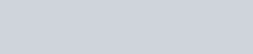# Atomic and Nuclear Phenomena

The Atomic and Nuclear Phenomena is part of the physics section provides High Yield information for the MCAT, USMLE, COMLEX, Medical School, Residency, and in the future career as a Physician. Prepare and Learn Ahead!

#### The Photoelectric Effect

• When light of a sufficiently high frequency is incident on a metal in a vacuum, the metal atoms emit electrons.
• Electrons emitted will produce a current. The higher the intensity of light, the greater the number of photons per unit time that fall on the electrode which in turn produces a larger current.

##### Threshold Frequency
• The minimum frequency of light that causes ejection of electrons. Depends on the type of metal.
• All-or-nothing response. Frequency of photon must be above threshold frequency for photoelectric effect to take place.
• If frequency is above, the radiation will have enough energy to dislodge the electrons in the metal, and the maximum kinetic energy of the ejected electron is equal to the difference between hf and hfT
• Photons: light beams consist of these integral number of light quanta. Energy is proportional to the frequency of the light:
• E = hp, h is Planck’s constant (6.626 x 10-34 J·s)
• Waves with higher frequency have shorter wavelengths and higher energy.

##### Kinetic Energy of Ejected Electrons
• If frequency of photon is more than threshold frequency, the electron will be ejected with kinetic energy:
• Kmax = hf – W
• W = hfT
• W is the work function and this is the minimum energy required to eject an electron.
• Only gives the maximum kinetic energy possible, this is only achieved when all possible energy from the photon is transferred completely to the electron (not possible).

#### Absorption and Emission of Light

• Relates to the Bohr model: An electron can jump from a lower energy level to a higher energy level by absorbing a photon of light with precisely the right frequency. Likewise, when an electron’s energy level is reduced, a photon is emitted.
• Infrared Spectroscopy is used to determine chemical structure since different bonds absorb different wavelengths
• UV-Vis Spectroscopy looks at both visible and UV light absorption.
• Fluorescence: if a fluorescent substance is excited by UV radiation, the substance will begin to glow with visible light.
• UV radiation causes electrons to be excited, substance returns to original state through two steps. The two steps allow for a smaller amount of energy to be used for each step and thus longer wavelength radiation to be emitted.

#### Nuclear Binding Energy and Mass Defect

• Mass Defect: difference between the theoretical mass of a nucleus (mass of protons + neutrons) and the actual mass. The actual mass is always smaller than the theoretical since some mass has been converted to the speed of light. Found from Einstein’s equation:
• E = mc^2
• Protons and neutrons are attracted to each other by the strong nuclear force, which is strong enough to overcome the repulsive force between protons.
• Only acts over extremely short distances, but is the strongest fundamental force
• Binding Energy: allows the nucleons to bind together in the nucleus. Caused by the bonded nucleons having a lower energy than the unbonded constituents. The difference in energy is emitted as some form of radiation.
• The higher the binding energy, the more stable the nucleus of the atom is. Intermediate sized nuclei are more stable than small and large ones.
• Weak Nuclear Force is one-millionth as strong as the strong nuclear force. The other two fundamental forces are electrostatic and gravitational forces.

#### Nuclear Reactions

• Isotopic notation is when elements are preceded by their atomic number as a subscript and mass number as a superscript.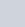• Atomic Number is the number of protons in the nucleus
• Mass number is the number of protons and neutrons in the nucleus
• Fusion: occurs when small nuclei combine to form a larger nucleus
• Occurs in the sun where 4 hydrogen nuclei fuse to form one helium nucleus
• Fusion plants are less common than fission plants, but usually use deuterium (hydrogen with one neutron) and lithium nuclei.
• Fission: large nucleus splits into smaller nuclei. Rarely occurs spontaneously, but can be induced through the absorption of low-energy neutrons.
• Power plants work by using fission reactions which release more neutrons that subsequently cause a chain-reaction of other fission reactions.

• Naturally occurring spontaneous decay of certain nuclei accompanied by the emission of specific particles.
• Should know how to answer three types of problems:
• The integer arithmetic of particle and isotope species
• The use of exponential decay curves and decay constant

##### Isotope Decay Arithmetic and Nucleon Conservation
• When parent nucleus, X, undergoes nuclear decay to form daughter nucleus Y, the balanced reaction is: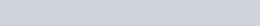• Sum of atomic numbers and mass numbers must be the same on both sides of the equation.

##### Alpha Decay
• The emission of an a-particle, which is a helium nucleus that consists of two protons and two neutrons.
• Massive particle (as compared to beta particle) and carries twice the charge.
• Interact with matter easily, so they do not penetrate shielding easily
•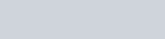##### Beta Decay
• The emission of a b-particle, which is an electron and is given the symbol e or b
• These are emitted along with antineutrino and a proton when a nucleus decays.
• Much more penetrating than alpha decay.
• Induced decay or positron emission can result in a positron being release, which is a particle with the same mass as the electron but has a positive charge.
• Neutrinos and antineutrinos are not tested on MCAT
•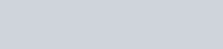##### Gamma Decay
• The emission of g-rays, which are high energy photons. Carry no charge and simply lower the energy of the parent nucleus.
•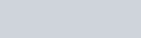##### Electron Capture
• Unstable radionuclides are capable of capturing inner electrons that combine with a proton to form a neutron, and that releases a neutrino.
• Make the atomic number one less than the original, but mass stays the same.
• Rare process and can be thought of as a reverse beta-negative decay.
•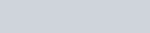##### Half-Life
• The time it takes for half of the sample to decay. (T1/2)

##### Exponential Decay
• If the rate of nuclei decay relates to the number of nuclei that remain, this suggests the following equation: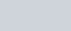• Solution of the above equation gives exponential decay: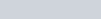•